## research papersBIOLOGICALCRYSTALLOGRAPHY
ISSN: 1399-0047
Volume 57| Part 10| October 2001| Pages 1451-1456

## Spherically averaged phased translation function and its application to the search for molecules and fragments in electron-density maps

aDepartment of Chemistry, University of York, Heslington, York YO1 5DD, England, and bSchool of Chemistry, University of Exeter, Stocker Road, Exeter EX4 4QD, England
*Correspondence e-mail: alexei@ysbl.york.ac.uk

(Received 9 February 2001; accepted 14 June 2001)

The molecular-replacement method has been extended to locate molecules and their fragments in an electron-density map. The approach is based on a new spherically averaged phased translation function. The position of the centre of mass of a search model is found prior to determination of its orientation. The orientation is subsequently found by a phased rotation function. The technique also allows superposition of distantly related macromolecules. The method has been implemented in a computer program MOLREP and successfully tested using experimental data sets.

### 1. Introduction

The molecular-replacement method (MR; Rossmann, 1972) is one of the principal techniques for determining the crystal structures of macromolecules. The location of a search model in the unit cell of the crystal of interest is usually divided into two three-dimensional searches. Firstly, the orientation of the model is found using a cross-rotation function (RF). Points in Eulerian space with high RF value indicate the probable orientations of a search molecule. The positional search is then carried out by applying the translation function (TF) to the search model orientated according to its highest RF values.

In spite of significant improvements in MR algorithms in recent years (Turkenburg & Dodson, 1996; Carter & Sweet, 1997), many cases remain unsolved for a variety of reasons. For some of these cases some prior phase information either from MIR/MAD or from a prepositioned partial structure may be available. Two approaches are currently used to locate molecules in an electron-density map. The phased translation function (PTF; Colman & Fehlhammer, 1976; Bentley, 1997) can be used to position the model in an unknown unit cell provided that the orientation of the search molecule is known. The disadvantage of this approach is that the conventional RF used to find the orientation of the model does not take into account prior phase information. Another approach is an exhaustive six-dimensional search of all possible orientations and positions of the search molecule in the electron-density map calculated either in direct space (ESSENS program; Kleywegt & Jones, 1997) or in reciprocal space (FFFEAR program; Cowtan, 1998). This six-dimensional search is computationally demanding even when a coarse grid is used.

We have extended the molecular-replacement method to locate macromolecules and their fragments in the electron density. The central point of the new approach is to position the centre of mass of the search model in the electron density prior to the determination of the model orientation. This is performed using a spherically averaged phased translation function (SAPTF). A local phased rotation function (PRF) is subsequently calculated to determine the orientation of the search molecule. A phased translation function (PTF) is used to check and refine the solution. This new approach also can be used for the superposition of distantly related macromolecules. The method was implemented in the program MOLREP and tested on a number of cases using experimental X-ray data sets.

### 2. Description of the method

Suppose that some prior phase information (experimental electron density) was available for a crystal of a biological macromolecule either from a MIR/MAD experiment or from a prepositioned partial structure and a search model is available which is either a homologous molecule or a molecular fragment (such as an α-helix). The suggested approach is to find the position of the centre of mass of the search model in the unit cell prior to determination of its orientation. This strategy is based upon the following idea. The electron density calculated for a search model can be spherically averaged within a certain sphere around its centre of mass to give its radial distribution. The experimental electron density can also be spherically averaged within a sphere of the same radius at each point of the unit cell. An overlap function between the two, i.e. the electron density for the model spherically averaged around its centre of mass and the experimental electron density spherically averaged within the same radius around a given point () in direct space, is referred to as spherically averaged phased translation function (SAPTF),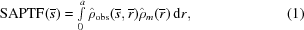where ρobs is the observed electron density, ρm is the electron density of the model,represents spherical averaging of the function to give its radial distribution,is the vector in direct space andis the origin of the new coordinate system.

The SAPTF therefore gives a maximum overlap for a correct position of the model. By expanding SAPTF into spherical harmonics it is possible to represent it as a Fourier series and to calculate it using fast Fourier transforms (Appendix A).

Once a putative centre of mass for a search model in the crystal has been located by SAPTF, its orientation at this position can be determined using a phased rotation function (PRF), which is defined as an overlap function between a rotated model electron density and an experimental electron density calculated within the same sphere. Its value has a maximum at a correct orientation for the model. This function is based on the fast rotation-function algorithm of Crowther (1972), but has the following modifications.

 (i) The calculations are conducted not in Patterson space but in real space using electron density. (ii) The centre of the integration sphere is the chosen centre of mass and is not necessarily at the origin. As the electron density does not possess a centre of symmetry, the expansion contains both odd and even spherical harmonics.
The model can be positioned in the experimental electron density using a suggested modification to molecular replacement in three steps. In step 1, a position for the centre of mass of a search model is determined in the electron density using SAPTF. High-scoring points of SAPTF indicate probable positions of centre of mass of a search molecule and are tabulated accordingly.

In step 2, for each highly scoring point of SAPTF a local PRF is calculated to find the orientation of the search molecule.

In step 3, the phased translation function is calculated for the orientations of the model found in step 2 to check and refine the position for the centre of mass that was found in step 1.

The above approach allows the separation of the rotation and translation searches. Since fast Fourier transforms are used to calculate the SAPTF and the PTF functions, this molecular-replacement search against electron density is almost as fast as classical molecular replacement. The most time-consuming part is calculating the PRF.

There is no need to have both the model and experimental electron densities averaged. An alternative approach to the one described above would be to spherically average only the model electron density. (1) can then be rewritten as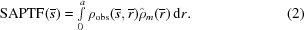This gives the possibility of performing the PTF search with the spherically averaged model electron density as a search model. This would require averaging of the electron density in real space and has not yet been implemented.

The SAPTF approach can also be used to superimpose distantly related macromolecules by fitting together the electron densities generated from the two sets of coordinates. The smaller of the two molecules is then taken to be the model and positioned against electron density generated from the larger molecule after placing it in a suitably large unit cell. This method of fitting of distantly related molecules has the following advantages.

 (i) There is no need to input information about possible matching pairs of atoms. (ii) The best fit between any two macromolecules can be determined, not just between two proteins. (iii) Proteins with no detectable sequence homology can be superimposed. (iv) The electron density from the model can be calculated at varying resolutions. This enables the desired level of detail to fit to be selected.

### 3. Applications

The SAPTF-based algorithm has been incorporated into the program MOLREP (Vagin & Teplyakov, 1997), a fully automated molecular-replacement program which utilizes an original full-symmetry TF combined with a packing function (Vagin, 1989).

The search for molecules and their fragments in electron density was tested on a number of cases and gave satisfactory results. Four of them are described here. The first test case illustrates a search for molecular fragments, namely α-helices, in the SIR density. The second test case is the positioning of a search model with no apparent sequence similarity to a crystallized protein into MIR electron density. The third test case shows the application of the SAPTF-based approach to superimpose two protein molecules which are not related in sequence or function but have similar folds. Test case 4 describes the superposition of non-protein macromolecules.

#### 3.1. Test 1. Positioning of a molecular fragment into isomorphous density

Hevamine from Hevea brasiliensis (PDB code 2hvm ; Terwisscha van Scheltinga et al., 1994) crystallizes in the orthorhombic space group P212121, with unit-cell parameters a = 52.3, b = 57.7, c = 82.1 Å. An isomorphous derivative was used for phasing to give SIR phases to 3 Å with an overall FOM of 0.45. The search model was a ten-residue α-helix. A SAPTF function (Fig. 1) was calculated with a radius of integration of 9 Å. Most of the SAPTF maximums are at the known helix positions and six helices of seven were correctly positioned by the procedure. This test case illustrates the necessity for including a relatively large number of possible SAPTF and PRF solutions in the calculations. The suggested algorithm seems to be more successful in searching for α-­helices than for β-­strands. We attribute this to the relative compactness of α-helices in comparison with elongated and flexible β-strands, which give poor contrast in SAPTF calculations.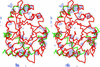Figure 1 Stereo diagram showing the SAPTF map contoured at 2.5σ (blue) calculated using a model of a ten-residue α-helix searched in the SIR electron density for hevamine (FOM = 0.45 to 3 Å) with a radius of averaging of 9 Å. The Cα trace of heavamine is shown in red. Cα traces of the model helices found by MOLREP using the SAPTF algorithm are shown in green, with most of the enzyme α-helices correctly identified. The figure was prepared using MOLSCRIPT (Kraulis, 1991).

#### 3.2. Test 2. The positioning of a distantly related model into isomorphous density

Esterase 713 from an Alcaligenes species (PDB code 1qlw ; Bourne et al., 2000) is a dimer of two identical sub­units of 318 residues each folded into a single domain with an α/β hydrolase fold. There is no detectable sequence similarity between esterase 713 and any other enzyme of known structure. The protein crystallized in the orthorhombic space group P212121, with unit-cell parameters a = 58.6, b = 116.8, c = 132.0 Å. The asymmetric unit contains an esterase dimer. MIR phases were derived from three heavy-atom derivatives to 3 Å with an overall FOM of 0.395. The search model was part of a monomer of acetylcholineesterase from Torpedo californica (PDB code 2ace ; Sussman et al., 1991) truncated to leave only the α/β hydrolase fold (230 residues of 527). The esterase 713 monomer and the search model superimpose with an r.m.s. deviation of 2.07 Å between their Cα positions over 159 residues. Conventional MR search with the model was conducted for a range of resolution limits and integration radii by both MOLREP (Vagin & Teplyakov, 1997) and AMoRe (Navaza, 1994). Both programs failed to find either the correct orientations for the search model or the translation solution for the correctly orientated model. However, a SAPTF-based search with MOLREP using MIR phases at 10–3 Å found the molecular-replacement solution for both subunits (Figs. 2a and 2b). The two correct solutions had the highest peak values for both SAPTF and PRF. The correct solutions displayed even better contrast when density-modified phases after NCS averaging were used.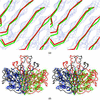Figure 2 Positioning of the α/β hydrolase fold part of acetylcholineesterase from T. californica onto 3 Å MIR electron density of esterase 713 from Alcaligenes species found by MOLREP using the SAPTF algorithm. The figure was prepared using MOLSCRIPT (Kraulis, 1991). (a) A stereo diagram of the Cα trace of acetylcholineesterase (green) positioned in the esterase 713 MIR electron density (blue) around a β-sheet of one of subunits. The Cα trace of the refined esterase model is shown in red. (b) A stereo diagram of the Cα trace of two molecules of acetylcholineesterase (green and blue) superimposed onto the Cα trace of refined esterase dimer; subunits are shown in red and black.

#### 3.3. Test 3. The suggested approach can also be used to superimpose distantly related molecules

In this case, a SAPTF-based search is conducted to determine the maximal overlap of electron densities calculated from atomic models rather than the best fit between the atomic positions as in most model superposition algorithms. Thermococcus litoralis pyrrolidone carboxyl peptidase (PDB code 1a2z ; Singleton et al., 1999) and Escherichia coli purine nucleotide phosphorylase (PDB code 1a69 ; Koellner et al., 1998) have similar folds but are not related by sequence or function. These two enzymes were superimposed by MOLREP (Fig. 3). From the three-dimensional alignment only it could be seen that the Cα backbones of the two models superimpose with an r.m.s. deviation of 1.9 Å over 119 residues.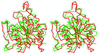Figure 3 A stereo diagram of the Cα trace of one subunit of pyrrolidone carboxyl peptidase (red) with the Cα trace of purine nucleotide phosphorylase (green) superimposed by MOLREP using the SAPTF algorithm. Figure prepared using MOLSCRIPT (Kraulis, 1991).

#### 3.4. Test 4. Superposition of non-protein macromolecules

The advantage of the suggested approach for the alignment of macromolecules is that the search models can differ in size and conformation and can be extended to other non-protein molecules. Fig. 4shows a superposition of the yeast Phe tRNA (PDB code 1tn2 ; Brown et al., 1983) with the Ser-2 tRNA from T. thermophilus from its complex with seryl-tRNA synthetase (PDB code 1ser ; Belrhali et al., 1994). Although the latter model is incomplete and there are conformational differences between the models, MOLREP correctly superimposed the common core.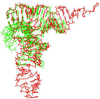Figure 4 A superposition of atomic models of the yeast Phe tRNA (green) and the Ser-2 tRNA from T. thermophilus from its complex with seryl-tRNA synthetase (red) found by MOLREP using the SAPTF algorithm at 100–4 Å resolution. Figure prepared using MOLSCRIPT (Kraulis, 1991).

### 4. Distribution

The program MOLREP is written in standard Fortran 77 and can be run under UNIX, Linux and Windows. The program MOLREP is available free from AAV, anonymous ftp account ftp.ysbl.york.ac.uk , http://www.ysbl.york.ac.uk/~alexei/ or from CCP4. Inquiries about the program should be addressed to AAV at alexei@ysbl.york.ac.uk.

### Spherically averaged phased translation function (SAPTF)

If we have an electron density ρ(), we can expand it within a spherical volume ra,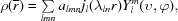where= r (r, υ, φ) in polar coordinates, jl is the spherical Bessel function of order l, λln zeroes the Bessel function such that jl (λlna) = 0 (n = 1, 2, …), a is the radius of the sphere (in the origin of coordinate system), Yml are the spherical harmonics and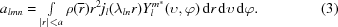For the new coordinate system with the origin at point,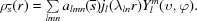The Fourier series corresponding to a crystal is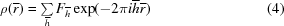and for a new origin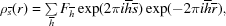where Fis the structure factor,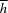(R, Y, Φ) is the vector in the polar coordinate system in reciprocal space andis the new origin of the coordinate system. Substituting (4) in (3),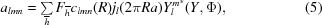where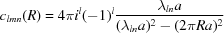and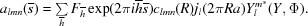Series with coefficients a00n, n = 1, 2, …, represent the spherically averaged function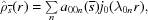whererepresents spherical averaging of the function and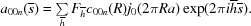For a model with its centre of gravity at the origin of the coordinate system,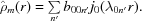The spherically averaged phased translation function (SAPTF) is defined as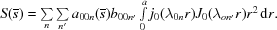The integral above is not 0 when n = n′,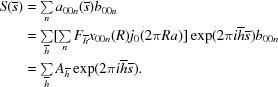The last expression is a Fourier series with coefficients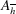=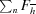c00n(R)j0(2πRa)b00n and can be calculated using the fast Fourier transform.

### Acknowledgements

We thank Garib Murshudov, Eleanor Dodson and Alexei Teplyakov for useful discussions and Ewald Schroder for helpful comments on the manuscript. AAV is supported by the Collaborative Computational Project, Number 4. MNI is supported by a postdoctoral fellowship from the BBSRC Chemical and Pharmaceutical Directorate.

### ReferencesBIOLOGICALCRYSTALLOGRAPHY